There are numerous exciting ways to divide numbers and cells in Excel.

Let’s discuss each of the following points regarding the Division in Excel:

### Using Division Formula/Operator

The most common way to divide in Excel is to use the Division operator i.e. the forward-slash symbol (/).

What does it do?

Divides two numbers

Formula breakdown:

=number1 / number2

What it means:

=the number being divided / the number you are dividing by

In Excel, you can divide two numbers very easily using the Division formula!

The Division Formula is done through the use of the division operator which is depicted by a forward slash:  /

I will show you in the steps below how you can divide numbers in Excel.

This is the table of values that we want to perform division on: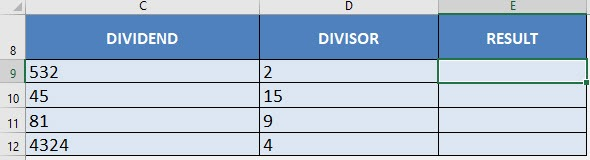I explain how you can do this below:STEP 1: We need to enter the number we want to divide:

## =C9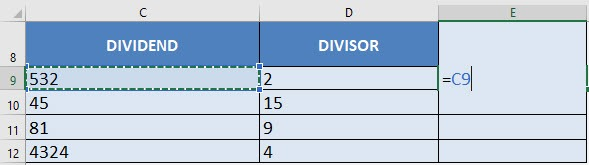STEP 2: Enter the division operator /

## =C9 /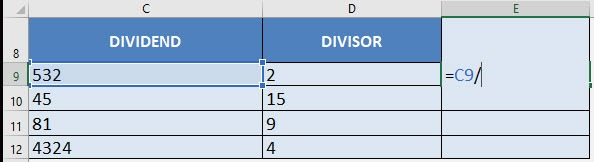STEP 3: Enter the number we are dividing by:

## =C9 / D9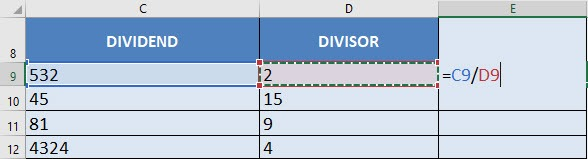Apply the same formula to the rest of the cells by dragging the lower right corner downwards.

You now have all of the division results!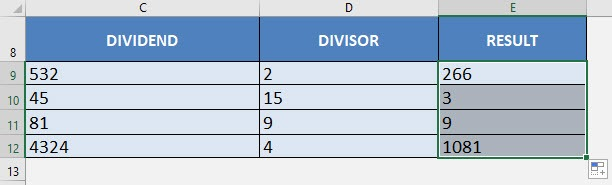### Divide by a Constant Number

In the example below, you have the total sales achieved each month by a company. You want to convert the sales amount in \$ ‘000 i.e. you want to divide each sales amount by 1000.

How can you quickly divide the sales amount by a constant value i.e. 1000?

Follow the step-by-step tutorial below to achieve the desired result:

STEP 1: Insert the divisor (i.e. 1000) in cell G6.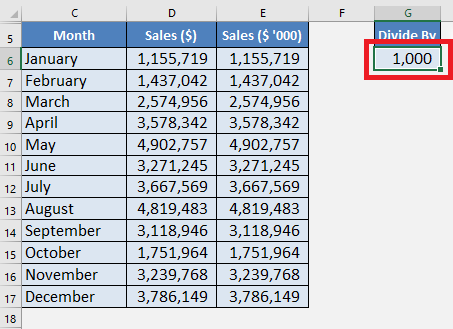STEP 2: Copy the value in cell G6. It will be saved in the clipboard.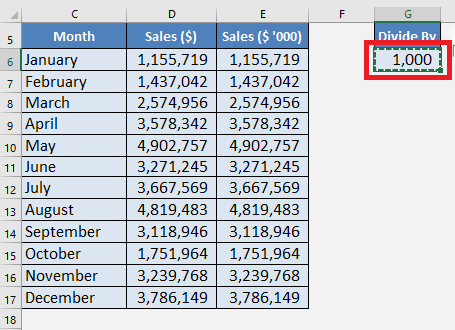STEP 3: Highlight the cells in Column E.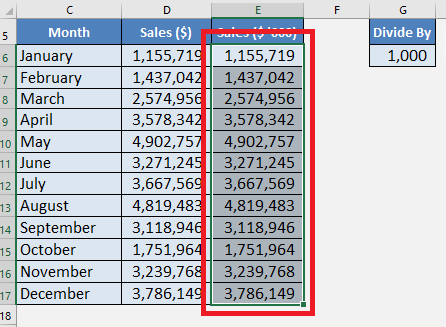STEP 4: Press Alt + E + S to open the Paste Special dialog box.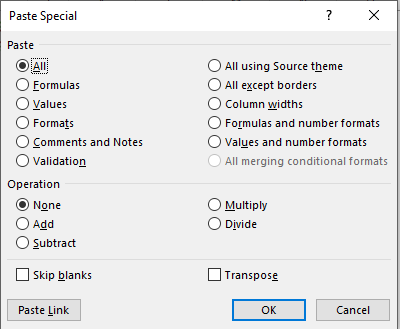STEP 5: Press to select the Divide option. Press OK.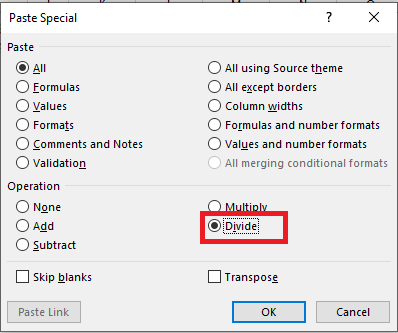The values in Column D is divided by 1000.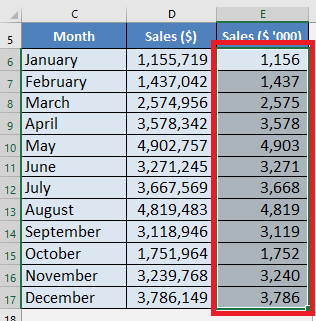So, you can easily divide the numbers by a constant value using the paste special option in Excel.

### Using Quotient Function

The Quotient Function is used to divide two numbers and return only the integer portion of the division. It does not provide the remainder and hence can be used when you want to omit the remainder.

If two numbers divide evenly without any remainder, then the result for both Division Operator (/) and Quotient function will be the same.

The Syntax of Quotient Function is:

=QUOTIENT(numerator, denominator)

where;

• Numerator – the dividend, i.e. the number to be divided.
• Denominator – the divisor, i.e. the number to divide by.

Make sure that the arguments mentioned in numerator and denominator are numeric, otherwise the QUOTIENT will return #VALUE! error.

Let’s take an example to see how this function work: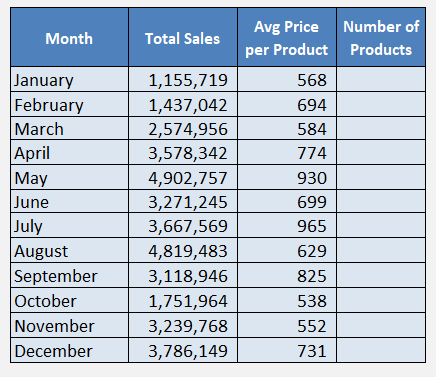Here, you have the total sales achieved by a company each month and the average sale price per product.

Now, you want to know the number of products sold each month!

This can be done using the Quotient Function to divide total sales by average price per product. As the result of this function will provide you with the integer portion of the division.

STEP 1: Select cell F6 and type = to start the formula.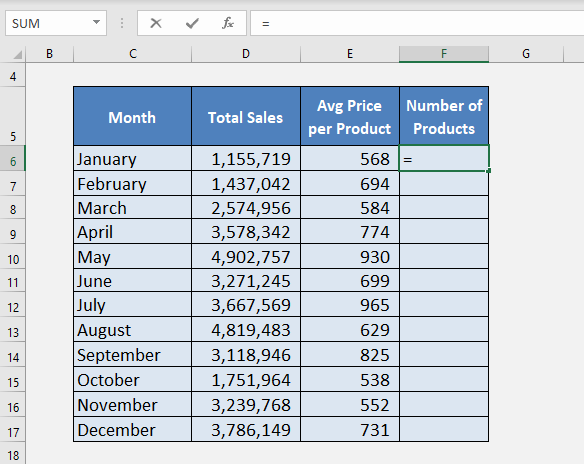STEP 2: Type Quotient and open bracket (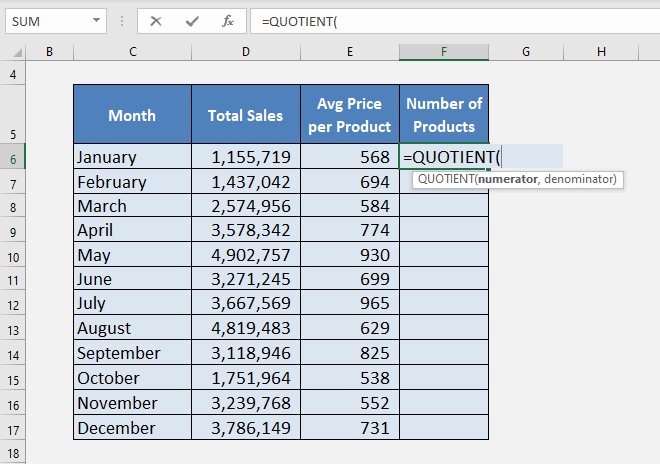STEP 3: Select Cell D6 to insert Total sales as the numerator and then type ,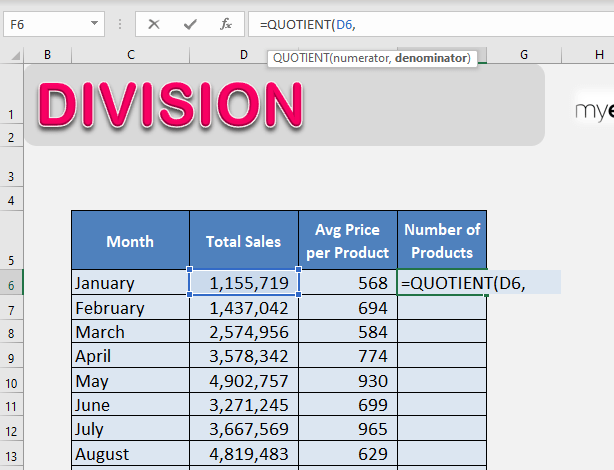STEP 4: Select Cell E6 to insert Average price per Product as a denominator and then enter close bracket ).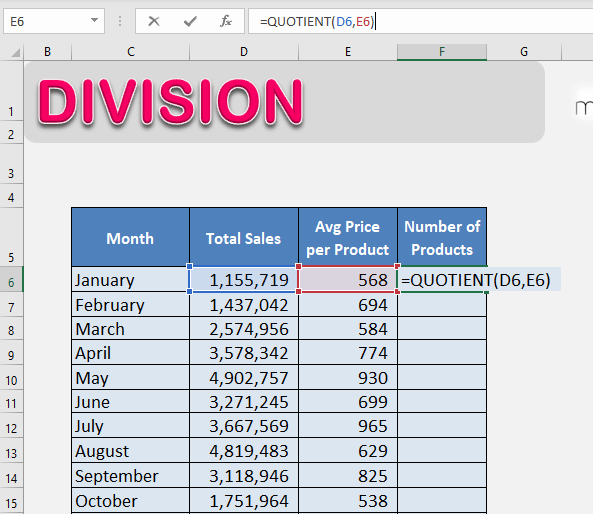STEP 5: Press Enter.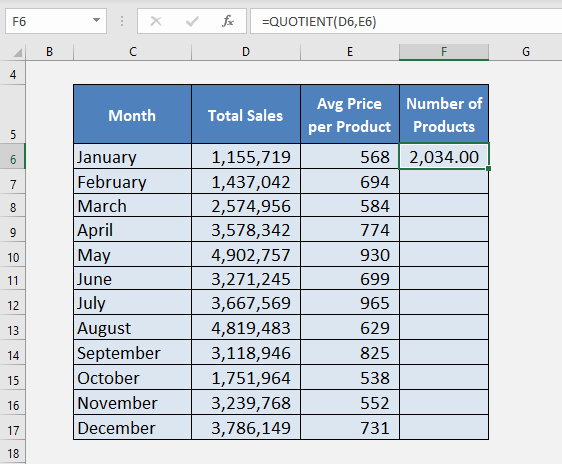This will provide you with only the integer portion of the division formula in Excel.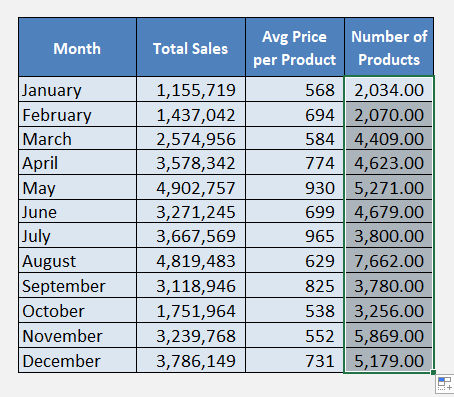### #DIV/0! Error in Excel Division Formula

Excel shows a #DIV/0! error when you try to divide a number by 0 or with a blank cell.

If you try a formula like =5/0, it will produce an error.

Look at the example below, when you tried to divide 6441 by 0 Excel has provided you with an #DIV/0! error.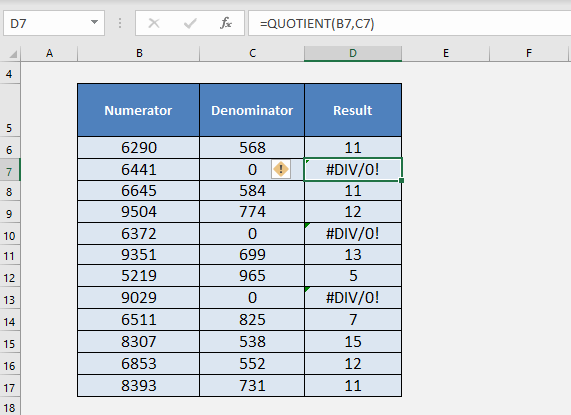You can easily replace this error with a custom text that you want using an IFERROR function.

IFERROR function returns a value you specify if a formula evaluates to an error; otherwise, it returns the result of the formula.

The syntax of IFERROR is =IFERROR(value,value_if_error)

• value – It is any value or cell reference or an expression.
• value_if_error – The text/value you want to display if the first argument returns an error.

Let’s use this function in our example, to replace the error with a blank.

You can use the formula =IFERROR(B7/C7,””)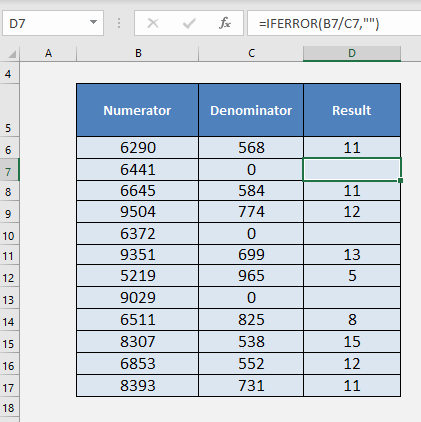The #DIV/0! error has been replaced!!

### Conclusion

In this article, you have been provided with a detailed guide on how to divide in Excel using the division operator and quotient function.

You can learn more about Excel formulas & functions and become better at Excel by clicking here.

Make sure to download our FREE PDF on the 333 Excel keyboard Shortcuts here:You can learn more about how to use Excel by viewing our FREE Excel webinar training on Formulas, Pivot Tables, and Macros & VBA!